# NCERT Solutions for Class 11 Economics Chapter 5 - Market Equilibrium

1. Explain market equilibrium.

Ans. In market equilibrium, the aggregate quantity that all firms wish to sell equals the quantity that all the consumers in the market wish to buy. In other words, market equilibrium is a situation where market supply equals market demand.

2. When do we say there is excess demand for a commodity in the market?

Ans. When the market demand of any product exceeds market supply at a particular price, then the situation is called excess demand.

3. When do we say there is excess supply for a commodity in the market?

Ans. If at a price, market supply is greater than market demand, we say that there is an excess supply in the market at that price.

4. What will happen if the price prevailing in the market is:
(i) above the equilibrium price?
(ii) below the equilibrium price?

Ans.

1. When price prevailing in the market is above the equilibrium price, demand will be less than supply, i.e., there is excess supply in the market.
2. When price prevailing in the market is below the equilibrium price, demand will be more than supply, i.e., there is excess demand in the market.

5. Explain how price is determined in a perfectly competitive market with fixed number of firms.

Ans. In a perfectly competitive market, equilibrium price is determined by the forces of market demand and market supply. Equilibrium price is the price at which the market demand becomes equal to market supply. If, at any particular price, demand and supply are equal, the buyers and sellers both remain satisfied, for at the said price the sellers supply what the buyers demand, and vice versa. Therefore, the buyers and sellers accept this price, and buy and sell accordingly.
That is why this price is called the equilibrium price.

6. Suppose the price at which equilibrium is attained in above question the minimum average cost of the firms constituting the market. Now if we allow for free entry and exit of firms, how will the market price adjust to it?

Ans. The price at which equilibrium attained in above question the minimum average cost of the firms constituting the market then it implies that the firm is earning supernormal profits. This situation attracts new firms to enter into the market hence industry supply of output also increases. New firms will continue to enter the industry that will lead the price to fall until it becomes equal to the minimum of the average cost. At this stage all the firms earn normal profits. When the free entry and exit of firms is allowed, the equilibrium is determined by the intersection of demand curve and the P = min AC line.

7. At what level of price do the firms in a perfectly competitive market, supply when free entry and exit is allowed in the market? How is equilibrium quantity determined in such a market?

Ans. In the long run, due to the free entry and exit of firms all the firms earn zero economic profit or normal profit. They neither earn abnormal profits nor abnormal losses. Thus the free entry and exit feature ensures that in the long run the equilibrium price will be equal to the minimum of average cost irrespective of whether profits or losses are earned in the short run. The equilibrium is determined by the intersection of consumers demand cruve and the P = min AC line.

8. How is the equilibrium number of firms determined in a market where entry and exit is permitted?

Ans. With the free entry and exit of firms in a perfect competitive market, the equilibrium number of firms can be determined by equilibrium quantity supply per firm. In this type of situation, no new firm is allowed to enter into the market or no existing firm will leave, if the price is equal to the minimum of LAC. Thus, the number of firms is determined by the equality of price and the minimum of LAC. The market equilibrium is determined by the intersection of market demand curve (D1D1) and the price line. The equilibrium price is P1 and the equilibrium output is q1. At this equilibrium price, each firm supplies the same output q1f , as it is assumed that all the firms are identical. Therefore, at the equilibrium, the number of firms in the market is equal to the number of firms required to supply output q1 at price P1, and each in turn supplying q1f amount at this price. That is
Where,
n = number of firms at market equilibrium
q1 = the equilibrium quantity demanded
q1f = the quantity of output supplied by each firm

9. How are equilibrium price and quantity affected when income of the consumers
(a) increase?
(b) decrease?

Ans. (a) Increase in income of consumers:If the number of firms is assumed to be fixed, when income of the consumer increase the demand will also increase because the consumer would pay higher price but the supply remains the same, so there will be a rise in equilibrium price. As a result of this, the demand curve will shift rightward.
Let us understand how it happens:
D1D1 and S1S1 represent the market demand and market supply respectively. The initial equilibrium occurs at E1, where the demand and the supply intersect each other. Due to the increase in consumers’ income, the demand curve will shift rightward parallelly while the supply curve will remain unchanged. Hence, there will be a situation of excess demand, equivalent to (qe – q1). Consequently, the price will rise due to excess demand. The price will continue to rise until it reaches E2 (new equilibrium), where D2D2 intersects the supply curve S1S1. The equilibrium price increases from Pe to P2 and the equilibrium output increases from qe to q2

(b) Decrease in the income of consumers: If the number of firms is assumed to be fixed, when income of the consumer decrease the equilibrium price will also decrease because the consumer is not in a condition to pay higher price but the supply remains the same. As a result of this, the demand curve will shift leftward.The decrease in consumers’ income is depicted by leftward parallel shift of demand curve from D1D1 to D2D2. Consequently, at the price Pe, there will be an excess supply (qe − q1), resulting the price to fall. At the new equilibrium (E2), where D2D2 intersects the supply curve, the equilibrium price falls from Pe to P2 and the equilibrium quantity falls from qe to q2

10. Using supply and demand curves, show how an increase in the price of shoes affects the price of a pair of socks and the number of pairs of socks bought and sold.

Ans. Shoes and socks are complementary goods. Accordingly, as the demand for socks is expected to fall with rise in the price of shoes thus the demand curve (for socks) shifts backward. When demand curve shifts backward, the equilibrium price and quantity will be affected as shown in Fig.In the above fig. D is the initial demand curve and S is the initial supply curve. E is the equilibrium point where D and S intersect each other. OP is the equilibrium price whereas OQ is the equilibrium quantity demanded of socks. When the price of shoes increases, demand curve for socks shifts backward as indicated by D1. Equilibrium price shifts from E to E1 and equilibrium quantity falls from OQ to OQ1.

11. How will a change in price of coffee affect the equilibrium price of tea? Explain the effect on equilibrium quantity also through a diagram.

Ans. Coffee and tea are substitute goods, i.e., they are used in place of each other. A change in price of coffee will directly influence the equilibrium price and quantity demanded of tea and vice-versa.

The figure given below depicts the equilibrium point of the tea market. The initial demand and supply curves of tea are depicted by D1D1 and S1S1 respectively. The initial equilibrium is at E1, with the equilibrium price (pe) and equilibrium quantity (qe). Now, if the price of coffee increases, the demand for the same decreases which will result to an increase in the demand for tea (being a substitute good) hence, the demand curve of tea will shift parallelly rightward and the price of tea will rise. At the equilibrium price (pe), there will be an excess demand for tea; consequently, the price of tea will rise. This will form the new equilibrium at E2, with the new equilibrium price increases from Pe to P2 and the new equilibrium output q2. Hence, an increase in the price of coffee, will lead the equilibrium price of tea to rise (due to the excess demand). Further, the increase in the price of coffee will also lead to the increase in demand for tea as tea is the substitute to coffee.

Now, if the price of coffee decreases, the demand for coffee increases and there will be a decrease in the demand for tea. The demand curve for tea will shift parallelly leftward to D2D2. At the equilibrium price (Pe), there will be an excess supply. Consequently, the price of tea will fall, which will form the new equilibrium at E2, with the new equilibrium price falls from Pe to P2 and the new equilibrium output decreases from qe to q2. Hence, a decrease in the price of coffee will lead to a decrease in the price of tea and a decrease in the demand for tea, as people will switch over to consumption of coffee.12. How do the equilibrium price and quantity of a commodity change when price of input used in its production changes?

Ans. A change in price of inputs will directly influence the equilibrium price and quantity of goods. When there is increase in the price of a commodity, will cause a decrease in the supply of that commodity and leads to a leftward shift of the supply curve as shown in the diagram given below. But the demand curve of the commodity remains the same and this will cause the market price of the commodity to rise and quantity demanded to fall.

It is clear from the diagram, that as a result of a decrease in supply, the supply curve shifts leftward. As a result, the price rises from OP to OP1 and the quantity falls from OQ to OQ113. If the price of a substitute(Y) of good X increases, what impact does it have on the equilibrium price and quantity of good X?

Ans. An increase in price of a substitute (Y) of good (X) will directly affect the equilibrium price and quantity of good (X). Changes in the price of one substitute good tends to change the demand for another substitute good. The demand for good X will increase due to the increase in the price of good Y. This situation causes an increase in the price of good X due to excessive demand.14. Compare the effect of shift in demand curve on the equilibrium when the number of firms in the market is fixed with the situation when entry-exit is permitted.

Ans. When the number of firms in the market is fixed. In this situation, Increase in demand will raise both equilibrium price and quantity. When entry and exit of firms in the market are permitted. Here, new firms will be attracted by super normal profits arising due to the excess demand (caused by an increase in demand). It will result in a fall in price till it becomes equal to minimum AC. Therefore, the equilibrium price remains unchanged. Decrease in demand will result in the fall of both equilibrium price and quantity.

15. Explain through a diagram the effect of a rightward shift of both the demand and supply curves on equilibrium price and quantity.

Ans. When both demand and supply of a commodity increase or shift to the right, the equilibrium quantity will increase but the equilibrium price may or may not be affected.

There may be three situations:

1. When both demand and supply of a commodity increase in equal proportions, the equilibrium price will remain the same. See Fig. (a).
2. When both demand and supply increase but the increase in demand is more than the increase in supply, the equilibrium price will rise. See Fig. (b).
3. When both demand and supply increase but the increase in demand is less than the increase in supply, the equilibrium price will fall. See Fig. (c).16. How are the equilibrium price and quantity affected when
(a) both demand and supply curves shift in the same direction?
(b) demand and supply curves shift in opposite directions?

Ans. (a)

 Cases Equilibrium Price Equilibrium Quantity Figure Increase in demand = Increase in supply No change IncreasesIncrease in demand more than increase in supply Increases Increases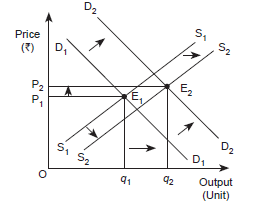Increase in demand less than increase in supply Decreases Increases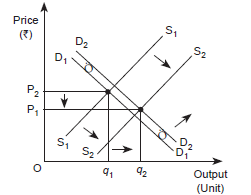Decrease in Demand = Decrease in Supply No change Decreases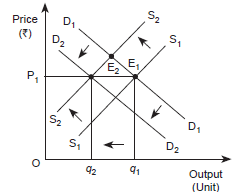Decrease in demand more than decrease in supply Decreases Decreases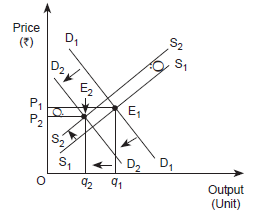Decrease in demand less than decrease in supply Increases Decreases(b)

 Cases Equilibrium Price Equilibrium Quantity Figure Increase in Demand = decrease in Supply Increases No change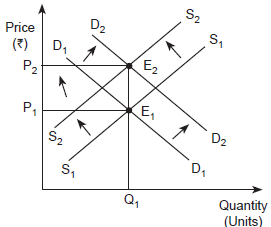Decrease in Demand = increase in Supply No change Increases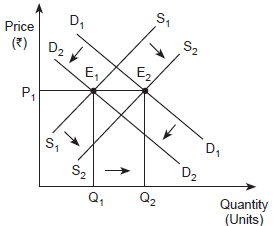Decrease in Demand is less than increase in supply Decreases Increases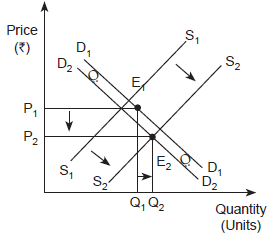Decrease in Demand is more than increase in supply Decreases Decreases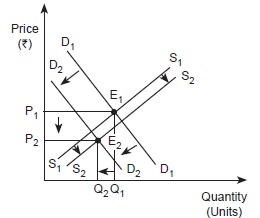Increase in Demand is less than decrease in supply Increases DecreasesIncrease in Demand is more than decrease in supply Increases Increases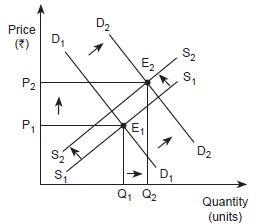17. In what respect do the supply and demand curves in the labour market differ from those in the goods market?

Ans. The supply and demand curves in the labour market differ from those in the goods market in the following ways:

1. In a goods market, the demand for goods is made by consumers or households; while in a labour market, the demand for labour is made by firms.
2. In a goods market, the supply of goods is made by firms; while in a labour market, the supply of labour is made by households.
So, in a goods market, firms act as suppliers; in a labour market, households act as suppliers.

18. How is the optimal amount of labour determined in a perfectly competitive market?

Ans. A profit maximizing firm will employ labour up to the point where the extra cost incurred by employing the last unit of labour (wage) equals the additional benefit it earns by employing that unit of labour.
That is, Marginal cost of labour = Marginal benefit by labour
or, W age rate = Marginal Revenue Product
or, w = MRPL
or, w = MR × MPL (as MRPL = MR × MPL)
or, w = P × MPL (in Perfect competition Price = MR)
or, w = VMPL (because VMPL = P × MPL

19. How is the wage rate determined in a perfectly competitive labour market?

Ans. Just like a goods market, wage rate in a labour market is determined by the intersection of demand and supply of labour. The rate at which the demand equals the supply is called the equilibrium wage rate. Corresponding hours of labour are demanded and supplied in the labour market at the equilibrium wage rate.

The demand for labour is derived from the value of marginal product of labour (VMPL). We know that a particular firm will employ labour up to a point where marginal cost of employing the last unit of labour hired equals the marginal benefit earned by the firm by hiring that unit of labour.
Labour is supplied by those households, who need to trade-off between working hours (labour) or leisure. The supply of labour is a positive function of wage up to a point beyond which the supply curve becomes backward bending supply curve.

20. Can you think of any commodity on which price ceiling is imposed in India? What may be the consequence of price-ceiling?

1. Ans. The government-imposed upper limit on the price of a good or service is called price ceiling. Price ceiling is generally imposed in India on necessary items like wheat, rice, kerosene, sugar etc.
The consequences of price-ceiling are as follows:
2. Each consumer has to stand in long queues to buy the good from ration shops.
3. Since all consumers will not be satisfied by the quantity of the goods that they get from the fair price shop, some of them will be willing to pay higher price for it. This may result in the creation of black market.
4. It has been found oftenly that the goods that are rationed are usually inferior goods and are adulterated.

21. A shift in demand curve has a larger effect on price and smaller effect on quantity when the number of firms is fixed compared to the situation when free entry and exit is permitted. Explain.

Ans. Under this condition, even if the market demand curve shifts in either direction, at the new equilibrium, the market will supply the desired quantity at the same price.

In the graph below, DD0 is the market demand curve which tells us how much quantity will be demanded by the consumers at different prices and P0 denotes the price which is equal to the minimum average cost of the firms. The initial equilibrium is at point E where the demand curve DD0 cuts the P0 = min AC line and the total quantity demanded and supplied is q0. The equilibrium number of firms is n0 in this situation.

Now suppose that the demand curve shifts to the right for some reason. At P0 there will be excess demand for the commodity. Some consumers will be willing to pay higher price for the commodity, so the price tends to rise. This gives rise to a possibility of earning super-normal profit which will attract new firms to the market. The entry of these new firms will eventually wipe out the super-normal profit and the price will again reach P0. Now, higher quantity will be supplied at the same price. From the panel (a), we can see that the new demand curve DD1 intersects the P0 = minAC line at point F such that the new equilibrium will be (P0, q1) where q1 is greater than q0. Similarly, for a leftward shift of the demand curve to DD2, there will be excess supply at the price P0. In response to this excess supply, some firms, which will be unable to sell their desired quantity at P0, will wish to lower their price. The price tends to decrease which will lead to the exit of some of the existing firms and the price will again reach P0. Therefore, in the new equilibrium, less quantity will be supplied which will be equal to the reduced demand at that price. This is shown in panel (b) where due to the shift of demand curve from DD0 to DD2, quantity demanded and supplied will decrease to q2 whereas the price will remain unchanged at P0. Thus, due to a shift in demand rightwards (leftwards), the equilibrium quantity and number of firms will increase (decrease) whereas the equilibrium price will remain unchanged.

Here, we should note that with free entry and exit, shift in demand has a larger effect on quantity demanded than it does with the fixed number of firms. But unlike with fixed number of firms, here, we do not have any effect on equilibrium price at all.22. Suppose the demand and supply curve of commodity X in a perfectly competitive market are given by:
qD = 700 – p
qS = 500 + 3p for p ≥ 15
= 0 for 0 ≤ p < 15
Assume that the market consists of identical firms. Identify the reason behind the market supply of commodity X being zero at any price less than ₹15. What will be the equilibrium price for this commodity? At equilibrium, what quantity of X will be produced?

Ans. It is given that;
qD = 700 − p
qS = 500 + 3p for p ₹15
= 0 for 0 ≤ p 15
The market supply is zero for any price from ₹0 to ₹15, this is because, for price between 0 to 15, no individual firm will produce any positive level of output (as the price is less than the minimum of AVC). Consequently, the market supply curve will be zero.
At equilibrium qD = qS
700 − p = 500 + 3p
− p −3p = 500 − 700
− 4p = − 200
p = 50
Equilibrium price is ₹50.
Quantity = qS = 500 + 3p
= 500 + 3 (50)
= 500 + 150
= 650 units
Therefore, the equilibrium quantity is 650 units.

23. Considering the same demand curve as in above question? Now let us allow for free entry and exit of the firms producing commodity X. Also assume that the market consists of identical firms producing commodity X. Let the supply curve of a single firm be explained as
qSf = 8 + 3p for p ≥ 20
= 0 for 0 ≤ p < 20
(a) What is the significance of p = 20?
(b) At what price will the market for X be in equilibrium? State the reason for your answer.
(c) Calculate the equilibrium quantity and number of firms.

Ans. qfs = 8 + 3 p for p ≥ ₹20
= 0 for 0 ≤ p < ₹20.
qd = 700 – p

1. For the price between 0 to 20, no firm is going to produce anything as the price in this range is below the minimum of LAC. So, at the price of ₹20, the price line is equal to the minimum of LAC.
2. As there is an existence of the freedom of entry and exit of firms, the minimum of AVC is at ₹20, also, the price of ₹20 is the equilibrium price. This is because in the long-run, all firms earn zero economic profit, which implies that the price of ₹20 is the equilibrium price and at any price lower than ₹20, the firm will move out of the market.
3. At equilibrium price of ₹20.
Quantity supplied = qs = 8 + 3p
= 8 + 3 (20)
qs = 68 units
Quantity demanded qd = 700 − p
= 700 − 20
qd = 680
Number of firms (n)
n=680/68
n = 10 firms
Therefore, the number of firms in the market is 10 and the equilibrium quantity is 680 units.

24. Suppose the demand and supply curves of salt are given by:
qD = 1,000 – p qS = 700 + 2p

1. Find the equilibrium price and quantity.
2. Now suppose that the price of an input used to produce salt has increased so that the new supply curve is
qS = 400 + 2>p
How does the equilibrium price and quantity change? Does the change conform to your expectation?
3. Suppose the government has imposed a tax of ₹3 per unit of sale of salt. How does it affect the equilibrium price and quantity?

Ans.

1. At equilibrium
qD = 1000 – p …(1)
qS = 700 + 2p …(2)
qD = qS
1000 − p = 700 + 2p
300 = 3p
100 = p
p = ₹100
qd = 1000-100
[Substituting the value of p in equation (1)]
= 900 units
So,
the equilibrium price is ₹100 and equilibrium quantity is 900 units.
2. New quantity supplied (qs) ...(3)
qs = 400 + 2p
At equilibrium qD = q′S
1000 − p = 400 + 2p
600 = 3p
200 = p
p = ₹200
Prior to the increase in the price of input, the equilibrium price was `100, and after the rise in input’s price, the equilibrium price is ₹200.
So,
the change in the equilibrium price is ₹100 (200 − 100).
qd = 1000 – P
= 1000 – 200 = 800 units
[Subtitling the value of p in equation (1)]
= 800 units
The change in the equilibrium quantity is 100 units (i.e., 900 − 800 units).
Yes, this change is obvious, as due to the change in the input’s price, the cost of producing salt has increased that will shift the marginal cost curve leftward and move the supply curve to the left. A leftward shift in the supply curve results in a rise in the equilibrium price and a fall in the equilibrium quantity.
3. The imposition of tax of ₹3 per unit of salt sold will raise the cost of producing salt. This will shift the supply curve leftwards and the quantity supplied equation will become
ys = 700 + 2(p − 3) ...(4)
At equilibrium
yd = ys
1000 − p = 700 + 2(p − 3)
1000 − p = 700 + 2p − 6
306 = 3p
p = ₹102
Substituting the value of p in equation (4)
yd = 1000 − p
yd = 1000 − 102
yd= 898 units
Thus, the imposition of tax of ₹3 per unit of salt sold will result in an increase in the price of salt from ₹100 to ₹102. The equilibrium quantity falls from 900 units to 898 units.

25. Suppose the market determined rent for apartments is too high for common people to afford. If the government comes forward to help those seeking apartments on rent by imposing control on rent, what impact will it have on the market for apartments?

Ans. In case the Government imposes price ceiling (means upper limit that a good or service cannot be charged over this price) that can be charged as rent of apartments results in decline in equilibrium price.The following figure shows the situation of price ceiling for apartments.
The market demand for apartments is depicted by the D1D1 curve and the supply of apartments is depicted by S1S1. The equilibrium price determined is R and the equilibrium quantity is q1.
If the government steps in and imposes rent ceiling (maximum rent) equivalent to RG, then at this rent, there will be an excess demand. The quantity of apartments demanded will be qd. Whereas, the quantity of apartments supplied is qs. So, there exists an excess demand equivalent to qd − qs. At the rate RG, common people can afford apartments to live in, which earlier they were not able to. However, besides this positive effect of imposition of maximum rent, it might happen that some landlords indulge in the practice of black marketing and offer apartments for rent at comparatively higher price.